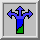# Fractal CoastlinesCoastline Applet

How is measuring the length of a coastline different from measuring the circumference of a circle or the distance around a square? For example: How long is the coastline along an ocean shore? Is its length equal to the mileage reading on the odometer of an automobile driving along the straight road beside the beach? Is it the distance covered by a bicyclist who pedals along the shore on a footpath? Is it the length run by a jogger whose morning exercise includes running ``next to the water?'' Is it the number of steps of a little bird running along the edge of the water looking for washed-up morsels? Whose ``length of coastline'' is the true one?

Trying to answer these innocent questions leads to deeper questions and our first look at natural fractals.

The following activities illustrates a strange property of a coastline: The measured length of a coastline depends on the length of the ruler you use. We will find that this and similar properties describe natural fractals.

Now explore these two hands-on activities. They will both help you understand what the Java applet is doing.

Now that you have an understanding of how a coastline is created (a model) and how a coastline can be measured, explore the Java applet and then answer the questions below.

Now that you've had a chance to experiment, can you answer these questions?

• Is there a quantitative difference between measuring the coastline by the "ruler method" and the "box method"? If so, can you explain why?

• What does it mean to you that an object has a fractional dimension? Why would scientists want to study such objects?